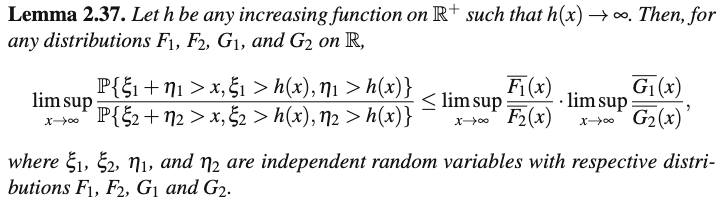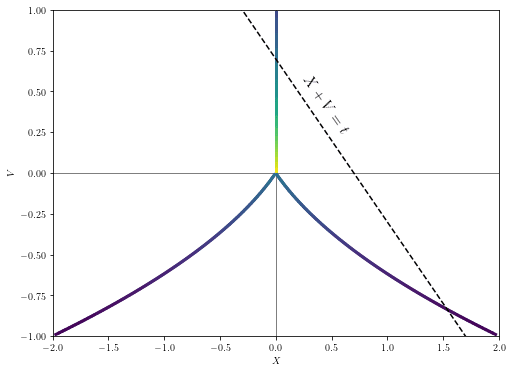# Catastrophic Regressional Goodhart: Appendix

10 min read15th May 20231 comment

# 10

Goodhart's LawWorld ModelingAI
Frontpage

This is a more technical followup to the last post, putting precise bounds on when regressional Goodhart leads to failure or not. We'll first show conditions under which optimization for a proxy fails, and then some conditions under which it succeeds. (The second proof will be substantially easier.)

# Related work

In addition to the related work sections of the previous post, this post makes reference to the textbook An Introduction to Heavy-Tailed and Subexponential Distributions, by Foss et al. Many similar results about random variables are present in the textbook, though we haven't seen this posts's results elsewhere in the literature before. We mostly adopt their notation here, and cite a few helpful lemmas.

# Main result: Conditions for catastrophic Goodhart

Suppose that  and  are independent real-valued random variables. We're going to show, roughly, that if

•  is subexponential (a slightly stronger property than being heavy-tailed).
•  has lighter tails than  by more than a linear factor, meaning that the ratio of the tails of  and the tails of  grows​​ superlinearly.

then .

Less formally, we're saying something like "if it requires relatively little selection pressure on  to get more of  and asymptotically more selection pressure on  to get more of , then applying very strong optimization towards  will not get you even a little bit of optimization towards  - all the optimization power will go towards ."

## Proof sketch and intuitions

The conditional expectation  is given by , and we divide the integral in the numerator into 4 regions, showing that each region's effect on the conditional expectation of V is similar to that of the corresponding region in the unconditional expectation .

The regions are defined in terms of a slow-growing function  such that the fiddly bounds on different pieces of the proof work out. Roughly, we want it to go to infinity so that  is likely to be less than  in the limit, but grow slowly enough that the shape of 's distribution within the interval  doesn't change much after conditioning.

In the table below, we abbreviate the condition  as .

Here's a diagram showing the region boundaries at , and  in an example where  and , along with a negative log plot of the relevant distribution:

Note that up to a constant vertical shift of normalization, the green curve is the pointwise sum of the blue and orange curves.

## Full proof

To be more precise, we're going to make the following definitions and assumptions:

• Let  be the PDF of  at the value . We assume for convenience that  exists, is integrable, etc, though we suspect that this isn't necessary, and that one could work through a similar proof just referring to the tails of . We won't make this assumption for .
• Let  and , similarly for  and
• Assume  has a finite mean:  converges absolutely.
•  is subexponential.
• Formally, this means that
• This happens roughly whenever  has tails that are heavier than  for any  and is reasonably well-behaved; counterexamples to the claim "long-tailed implies subexponential" exist, but they're nontrivial to exhibit.
• Examples of subexponential distributions include log-normal distributions, anything that decays like a power law, the Pareto distribution, and distributions with tails asymptotic to  for any .
• ​We require for  that its tail function is substantially lighter than X's, namely that for some .
• This implies that .

With all that out of the way, we can move on to the proof.

The unnormalized PDF of  conditioned on  is given by . Its expectation is given by .

Meanwhile, the unconditional expectation of V is given by  .

We'd like to show that these two expectations are equal in the limit for large . To do this, we'll introduce . (More pedantically, this should really be , which we'll occasionally use where it's helpful to remember that this is a function of .)

For a given value of  is just a scaled version of , so the conditional expectation of  is given by . ​But because , the numerator and denominator of this fraction are (for small ) close to the unconditional expectation and , respectively.

We'll aim to show that for all  we have for sufficiently large  that  and , which implies (exercise) that the two expectations have limiting difference zero. But first we need some lemmas.

## Lemmas

Lemma 1: There is  depending on  such that:

• (a)
• (b)
• (c)
• (d)  .

Proof: Lemma 2.19 from Foss implies that if  is long-tailed (which it is, because subexponential implies long-tailed), then there is  such that condition (a) holds and  is -insensitive; by Proposition 2.20 we can take  such that  for sufficiently large , implying condition (b). Conditions (c) and (d) follow from being -insensitive.

Lemma 2: Suppose that  is whole-line subexponential and  is chosen as in Lemma 1. Also suppose that . Then

Proof: This is a slight variation on lemma 3.8 from , and follows from the proof of Lemma 2.37. Lemma 2.37 states thatbut it is actually proved that

If we let , then we get

where . Multiplying by , we have ,

and because  as  and , we can say that for some . Therefore for sufficiently large t

By Theorem 3.6,  is , so the LHS is  as desired.

## Bounds on the numerator

We want to show, for arbitrary , that  in the limit as . Since

it will suffice to show that the latter quantity is less than  for large .

We're going to show that  is small by showing that the integral gets arbitrarily small on each of four pieces: , and .

We'll handle these case by case (they'll get monotonically trickier).

### Region 1: (−∞,−h(t)]

Since  is absolutely convergent, for sufficiently large  we will have, since  goes to infinity by Lemma 1(a).

Since  is monotonically increasing and , we know that in this interval .

So we have

as desired.

### Region 2: (−h(t),h(t))

By lemma 1(d),  is such that for sufficiently large  on the interval . (Note that the value of this upper bound depends only on  and , not on  or .) So we have

.

### Region 3: [h(t),t−h(t)]

For the third part, we'd like to show that . Since

it would suffice to show that the latter expression becomes less than  for large , or equivalently that  .

The LHS in this expression is the unconditional probability that  and ,​ but this event implies , and . So we can write

by Lemma 2.

### Region 4: (t−h(t),∞)

For the fourth part, we'd like to show that  for large .

Since , it would suffice to show . But note that since  by Lemma 1(c), this is equivalent to , which (by Lemma 1(b)) is equivalent to .

Note that , so it will suffice to show that both terms in this sum are

The first term  is  because we assumed  for some .

For the second term, we have for the same reason.

This completes the bounds on the numerator.

## Bounds on the denominator

For the denominator, we want to show that , so it'll suffice to show  as .

Again, we'll break up this integral into pieces, though they'll be more straightforward than last time. We'll look at , and .

• .
• But since  goes to infinity, this left tail of the integral will contain less and less of 's probability mass over time.

• But by Lemma 1(d) we know that this goes to zero for large .
• But for sufficiently large  we have , so we obtain

by the results of the previous section.

This completes the proof!

# Light tails imply V

Conversely, here's a case where we do get arbitrarily high  for large . This generalizes a consequence of the lemma from Appendix A of Scaling Laws for Reward Model Overoptimization (Gao et al., 2022), which shows this result in the case where  is either bounded or normally distributed.

Suppose that  satisfies the property that . This implies that  has tails that are dominated by  for any , though it's a slightly stronger claim because it requires that  not be too "jumpy" in the decay of its tails.

We'll show that for any  with a finite mean which has no upper bound,

In particular we'll show that for any

### Proof

Let , which exists by our assumption that  is unbounded.

Let . (If this is undefined because the conditional has probability , we'll have the desired result anyway since then  would always be at least .)

Observe that for all  (assuming it is defined), because we're conditioning  on an event which is more likely for larger  (since  and  are independent).

First, let's see that . This ratio of probabilities is equal to

which, by our assumption that , will get arbitrarily small as  increases for any positive .

Now, consider . We can break this up as the sum across outcomes  of  for the three disjoint outcomes , and . Note that we can lower bound these expectations by  respectively. But then once  is large enough that ,  this weighted sum of conditional expectations will add to more than  (exercise).

# Answers to exercises from last post

1. Show that when  and  are independent and . Conclude that . This means that given independence, optimization always produces a plan that is no worse than random.

Proof: Fixing a value of , we have for all  that. Since the conditional expectation after seeing any particular value of  is at least , this will be true when averaged across all  proportional to their frequency in the distribution of . This means that  for all , so the inequality also holds in the limit.

2. When independence is violated, an optimized plan can be worse than random, even if your evaluator is unbiased. Construct a joint distribution  for  and  such that , and  for any , but .

Solution: Suppose that  is distributed with a PDF equal to , and the conditional distribution of  is given by

where  is a random variable that is equal to  with 50/50 odds. The two-dimensional heatmap looks like this:Now, conditioning on  with , we have one of two outcomes: either , or  and
The first case has an unconditional probability of , and a conditional expectation of .
The second case has an unconditional probability of , and a conditional expectation of at most  since all values of  in the second case are at most that large.
So, abbreviating these two cases as  and  respectively, the overall conditional expectation is given by

as desired.

This sort of strategy works for any fixed distribution of , so long as the distribution is not bounded below and has finite mean; we can replace  with some sufficiently fast-growing function to get a zero-mean conditional  distribution that behaves the same.

For a followup exercise, construct an example of this behavior even when all conditional  distributions have variance at most .

1. ^

We actually just need , so we can have e.g. .

2. ^

We'll generally omit  and  terms in the interests of compactness and laziness; the implied differentials should be pretty clear.

3. ^

The diagrams in the previous post show visually that when  and  are both heavy-tailed and  is large, most of the probability mass has  or vice versa.

4. ^

This proof will actually go through if we just have  for any constant , which is a slightly weaker condition (just replace  with  in the proof as necessary). For instance,  could have probability  of being equal to  for , which would satisfy this condition for  but not

5. ^

If  has really jumpy tails, the limit of the mean of the conditional distribution may not exist. Exercise: what goes wrong when  has a  probability of being equal to  for  and  is a standard normal distribution?

# 10

Mentioned in
New Comment
1 comment, sorted by Click to highlight new comments since:

Prediction market for whether someone will strengthen our results or prove something about the nonindependent case: